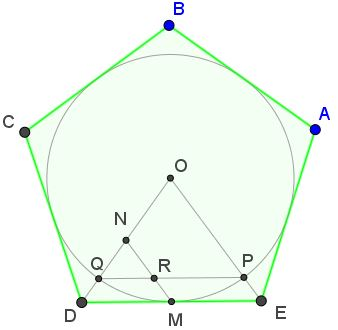# Golden Ratio in Regular Pentagon

The golden ratio, $\phi=\displaystyle\frac{1+\sqrt{5}}{2},$ makes frequent and often unexpected appearance in geometry. Regular pentagon - the pentagram - is one of the places where the golden ratio appears in abundance.To mention a few (some of which have been proved elsewhere, others are straightforward):

$\displaystyle\frac{DE}{EX}=\frac{EX}{XY}=\frac{UV}{XY}=\frac{EY}{EX}=\frac{BE}{AE}=\phi.$

Most recently Dao Thanh Oai posted an observation at the CutTheKntMath facebook page that in the following diagram $\displaystyle\frac{FB}{FA}=\phi ,$for $EF\perp DE.$ An easy proof is obtained by angle chasing. Observe that $\angle AED=108^{\circ},$ making $\angle AEF=18^{\circ}.$ Now draw $EB.$Note that $\angle BEF=18^{\circ}$ also, from which $EF$ is the bisector of $\angle AEB.$ Using the property of angles bisectors, $\displaystyle\frac{BF}{AF}=\frac{BE}{AE}=\phi.$

If $F'$ is t he intersection of $DE$ and $AB,$ then also $\displaystyle\frac{BF'}{AF'}=\phi,$ since $EF'$ is the external angle bisector in $\Delta ABE.$Further, there are multiple additional occurences of the Golden Ratio. For example, in the diagramwe have

$\displaystyle\frac{EF+CF}{DF}= \frac{FA + FD}{FE} = \frac{FC - FA}{FB},$

where $F\;$ may very widely.

Tran Quang Hung came up with an overlooked occurence of the Golden Ratio in a regular pentagon:Then, $\displaystyle\frac{ON}{QN}=\frac{PR}{QR}=\varphi.\;$ The details are in a separate page.### Golden Ratio